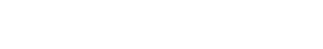# Floating Point Representation

## Introduction

In this article we are going to see what is a floating point and how decimal values are represented using this system.

Floating point representation is a way of writing a decimal number that resembles scientific notation. This allows us to represent and operate with very large numbers and also with very small numbers (with many decimals).

The floating point computing standard is described in IEEE 754.

## Structure of a floating point number

This representation system uses a certain number of binary digits depending on the type of accuracy (commonly 16, 32, 64 and 128 bits).

A bit is destined to the sign, i.e. if that bit is worth 0 it is a positive number, if it is worth 1 it is a negative number.

The remaining bits are distributed in the representation of the decimals (usually called mantissa) and the exponent.In the expression n it is the decimal number to represent.

The letter s is the bit for the sign (if s is 0, the expression (-1) raised to 0 results in 1 positive).

The letter e is the exponent and m is the mantissa.

## Move decimal numbers to floating point

1- Take the number to represent, separate the sign and write the absolute value in base 2.

2- The absolute value in base 2 is written in scientific notation in normalized base 2.

3- The exponent is expressed in excess notation (it will depend on the type of precision chosen).

4- The coefficient is written on the mantissa without the whole part, because the normalization in step 2 forces the whole part of the mantissa to be 1, storing it does not provide information.

## System Truncation Error Floating Point

A truncation error occurs when you take a certain number of digits from one number and leave out the others.

Think of the number π (3.14159265…), which is an irrational number with infinite digits.

Computers cannot store infinite information in memory because infinitely large memory would be needed, so at some point it must stop.

If we truncate all the decimal part to π and we are left with only 3, we will be making an error of approximately 4.5% relative to the real value of π . If on the other hand we take into account the first two decimals of π, we are left with 3.14. In this case we will be making an error of approximately 0.05% relative to the real value of π.

This error will occur in the floating point system either because we want to represent irrational numbers or because the decimal we want to represent becomes irrational when passed to the binary system (example 0.1 decimal has infinite digits when passed to binary).